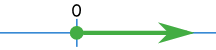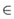# Composition of Functions

"Function Composition" is applying one function to the results of another:The result of f() is sent through g()

It is written: (g º f)(x)

Which means: g(f(x))

### Example: f(x) = 2x+3 and g(x) = x2

"x" is just a placeholder. To avoid confusion let's just call it "input":

f(input) = 2(input)+3

g(input) = (input)2

Let's start:

(g º f)(x) = g(f(x))

First we apply f, then apply g to that result:(g º f)(x) = (2x+3)2

What if we reverse the order of f and g?

(f º g)(x) = f(g(x))

First we apply g, then apply f to that result:(f º g)(x) = 2x2+3

We get a different result!

When we reverse the order the result is rarely the same.

So be careful which function comes first.

## Symbol

The symbol for composition is a small circle:

(g º f)(x)

It is not a filled in dot: (g · f)(x), as that means multiply.

## Composed With Itself

We can even compose a function with itself!

### Example: f(x) = 2x+3

(f º f)(x) = f(f(x))

First we apply f, then apply f to that result:(f º f)(x) = 2(2x+3)+3 = 4x + 9

We should be able to do it without the pretty diagram:

(f º f)(x)= f(f(x))
= f(2x+3)
= 2(2x+3)+3
= 4x + 9

## Domains

It has been easy so far, but now we must consider the Domains of the functions.The domain is the set of all the values that go into a function.

The function must work for all values we give it, so it is up to us to make sure we get the domain correct!

### Example: the domain for √x (the square root of x)

We can't have the square root of a negative number (unless we use imaginary numbers, but we aren't), so we must exclude negative numbers:

The Domain of √x is all non-negative Real Numbers

On the Number Line it looks like:Using set-builder notation it is written:

{ x| x ≥ 0}

Or using interval notation it is:

[0,+∞)

It is important to get the Domain right, or we will get bad results!

## Domain of Composite Function

We must get both Domains right (the composed function and the first function used).

When doing, for example, (g º f)(x) = g(f(x)):

• Make sure we get the Domain for f(x) right,
• Then also make sure that g(x) gets the correct Domain

### Example: f(x) = √x and g(x) = x2

The Domain of f(x) = √x is all non-negative Real Numbers

The Domain of g(x) = x2 is all the Real Numbers

The composed function is:

(g º f)(x) = g(f(x))
= (√x)2
= x

Now, "x" normally has the Domain of all Real Numbers ...

... but because it is a composed function we must also consider f(x),

So the Domain is all non-negative Real Numbers

## Why Both Domains?

Well, imagine the functions are machines ... the first one melts a hole with a flame (only for metal), the second one drills the hole a little bigger (works on wood or metal):What we see at the end is a drilled hole, and we may think "that should work for wood or metal". But if we put wood into g º f then the first function f will make a fire and burn everything down!

So what happens "inside the machine" is important.

## De-Composing Function

We can go the other way and break up a function into a composition of other functions.

### Example: (x+1/x)2

That function can be made from these two functions:

f(x) = x + 1/x

g(x) = x2

And we get:

(g º f)(x) = g(f(x))
= g(x + 1/x)
= (x + 1/x)2

This can be useful if the original function is too complicated to work on.

## Summary

• "Function Composition" is applying one function to the results of another.
• (g º f)(x) = g(f(x)), first apply f(), then apply g()
• We must also respect the domain of the first function
• Some functions can be de-composed into two (or more) simpler functions.